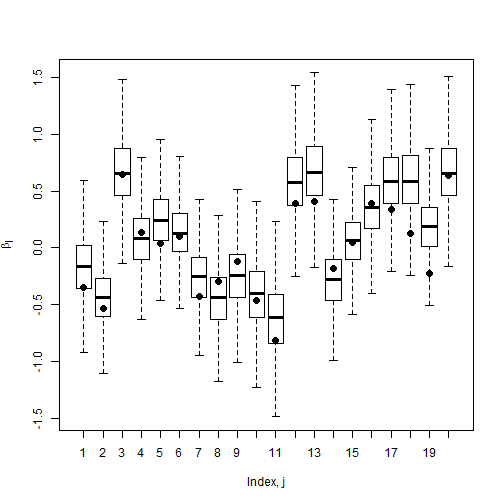# Metropolis + Gibbs sampling for simulated data

### Chapter 3.2.3: Metropolis sampling

Here we use simulate data to verify that the code is working properly. We generate a synthetic dataset and run MCMC to estimate the parameters. Unlike a real data analysis, for a simulation study we know the true values of the parameters and so this provides a check that the code is functioning well.

The data are generated from the logistic regression model $Y_i\sim\mbox{Bernoulli}(p_i) \mbox{ where } p_i = \frac{1}{1+\exp(-\sum_{j=1}^pX_{ij}\beta_j)}.$ The covariates are generated as $$X_{i1}=1$$ for the intercept and $$X_{ij}\sim\mbox{Normal}(0,1)$$ for $$j>1$$. The true values of $$\beta_j$$ are also sampled from a normal distribution.

We fit the model with prior $$\beta_j\sim\mbox{Normal}(0,\sigma^2)$$ and $$\sigma^2\sim\mbox{Gamma}(a,b)$$. The MCMC code using Metropolis steps to update the $$\beta_j$$ and a Gibbs step to update $$\sigma^2$$.

## Simulate data

 n          <- 100
p          <- 20
X          <- cbind(1,matrix(rnorm(n*(p-1)),n,p-1)) # first column for the intercept
true_beta  <- rnorm(p,0,.5)
prob       <- 1/(1+exp(-X%*%true_beta))
Y          <- rbinom(n,1,prob)


## Initialize

 # Create matrix to store the samples

S                 <- 25000
samples           <- matrix(NA,S,p+1)

# Initial values

beta   <- rep(0,p)
sigma  <- 1

# priors:

a      <- 0.1
b      <- 0.1

# candidate standard deviation:

can_sd <- 0.1


## Define the log posterior as a function

 log_post_beta <- function(Y,X,beta,sigma){
prob  <- 1/(1+exp(-X%*%beta))
like  <- sum(dbinom(Y,1,prob,log=TRUE))
prior <- dnorm(beta,0,10,log=TRUE) +        # Intercept
sum(dnorm(beta[-1],0,sigma,log=TRUE)) # Slopes
return(like+prior)}


## Metropolis sampling

 for(s in 1:S){

# Metropolis for beta
for(j in 1:p){
can    <- beta
can[j] <- rnorm(1,beta[j],can_sd)
logR   <- log_post_beta(Y,X,can,sigma)-
log_post_beta(Y,X,beta,sigma)
if(log(runif(1))<logR){
beta <- can
}
}

# Gibbs for sigma
sigma <- 1/sqrt(rgamma(1,(p-1)/2+a,sum(beta[-1]^2)/2+b))

samples[s,] <- c(beta,sigma)
}


## Plot the results

The boxplots are the posterior distributions for the $$\beta_j$$, and the points are the true values used to generate the data. The posteriors include the truth for most of the parameters indicating the alorithm is working well.

  boxplot(samples[,1:p],outline=FALSE,xlab="Index, j",ylab=expression(beta[j]))
points(true_beta,pch=19,cex=1.25)